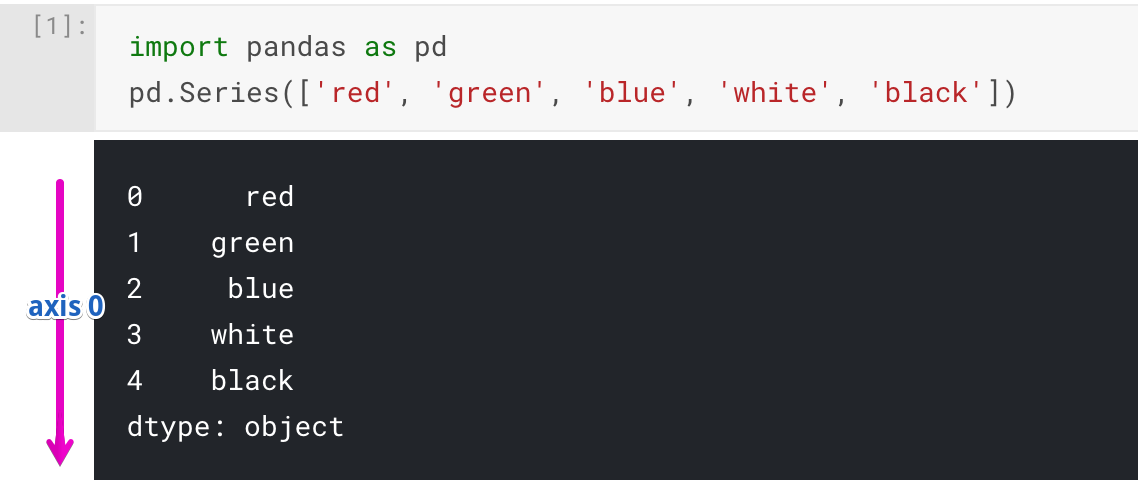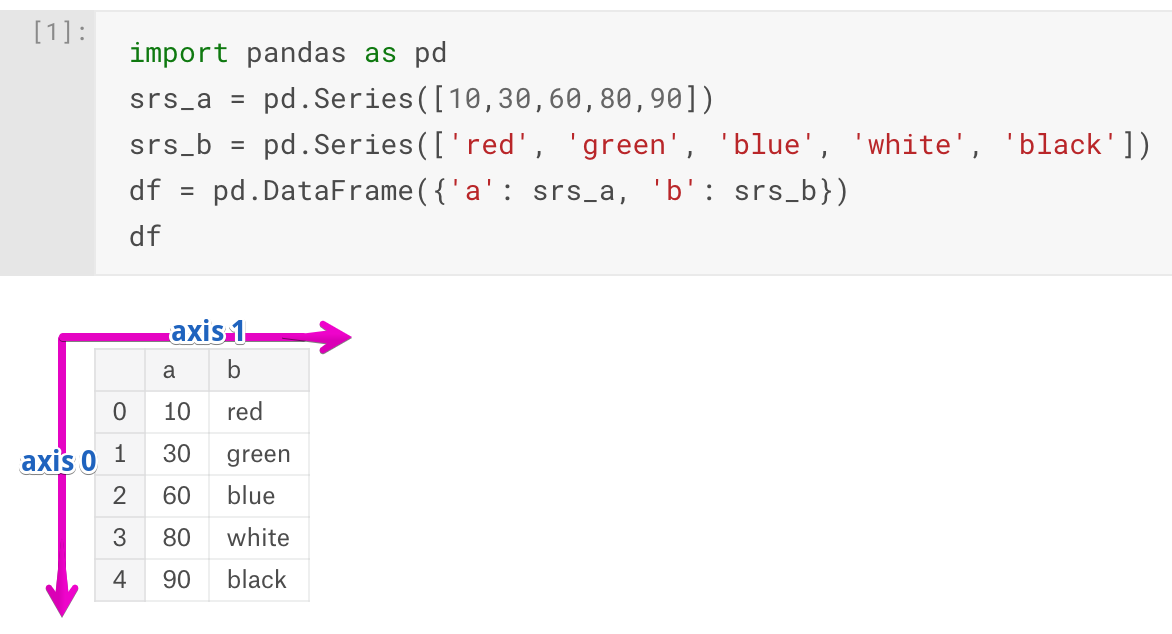## Python for Machine Learning: Pandas Axis ExplainedPandas is a powerful library in a toolbox for every Machine Learning engineer. It provides two main data structures: Series and DataFrame.

Many API calls of these types accept cryptical “axis” parameter. This parameter is poorly described in Pandas’ documentation, though it has a key significance for using the library efficiently. The goal of the article is to fill in this gap and to provide a solid understanding of what the “axis” parameter is and how to use it in various use cases including leading-edge artificial intelligence applications.

## Axis in Series

Series is a one-dimensional array of values. Under the hood, it uses NumPy ndarray. That is where the term “axis” came from. NumPy uses it quite frequently because ndarray can have a lot of dimensions.

Series object has only “axis 0” because it has only one dimension.The arrow on the image displays “axis 0” and its direction for the Series object.

Usually, in Python, one-dimensional structures are displayed as a row of values. On the contrary, here we see that Series is displayed as a column of values.

Each cell in Series is accessible via index value along the “axis 0”. For our Series object indexes are: 0, 1, 2, 3, 4. Here is an example of accessing different values:

```>>> import pandas as pd
>>> srs = pd.Series(['red', 'green', 'blue', 'white', 'black'])
>>> srs
'red'
>>> srs
'white'
```

## Axes in DataFrame

DataFrame is a two-dimensional data structure akin to SQL table or Excel spreadsheet. It has columns and rows. Its columns are made of separate Series objects. Let’s see an example:A DataFrame object has two axes: “axis 0” and “axis 1”. “axis 0” represents rows and “axis 1” represents columns. Now it’s clear that Series and DataFrame share the same direction for “axis 0” – it goes along rows direction.

Our DataFrame object has 0, 1, 2, 3, 4 indexes along the “axis 0”, and additionally, it has “axis 1” indexes which are: ‘a’ and ‘b’.

To access an element within DataFrame we need to provide two indexes (one per each axis). Also, instead of bare brackets, we need to use .loc method:

```>>> import pandas as pd
>>> srs_a = pd.Series([1,3,6,8,9])
>>> srs_b = pd.Series(['red', 'green', 'blue', 'white', 'black'])
>>> df = pd.DataFrame({'a': srs_a, 'b': srs_b})
>>> df.loc[2, 'b']
'blue'
>>> df.loc[3, 'a']
8
```

## Using “axis” parameter in API calls

There are a lot of different API calls for Series and DataFrame objects which accept “axis” parameter. Series object has only one axis, so this parameter always equals 0 for it. Thus, you can omit it, because it does not affect the result:

```>>> import pandas as pd
>>> srs = pd.Series([1, 3, pd.np.nan, 4, pd.np.nan])
>>> srs.dropna()
0    1.0
1    3.0
3    4.0
dtype: float64
>>> srs.dropna(axis=0)
0    1.0
1    3.0
3    4.0
dtype: float64
```

On the contrary, DataFrame has two axes, and “axis” parameter determines along which axis an operation should be performed. For example, .sum can be applied along “axis 0”. That means, .sum operation calculates a sum for each column:

```>>> import pandas as pd
>>> srs_a = pd.Series([10,30,60,80,90])
>>> srs_b = pd.Series([22, 44, 55, 77, 101])
>>> df = pd.DataFrame({'a': srs_a, 'b': srs_b})
>>> df
a    b
0  10   22
1  30   44
2  60   55
3  80   77
4  90  101
>>> df.sum(axis=0)
a    270
b    299
dtype: int64
```

We see, that having sum with axis=0 smashed all values along the direction of the “axis 0” and left only columns(‘a’ and ‘b’) with appropriate sums.

With axis=1 it produces a sum for each row:

```>>> df.sum(axis=1)
0     32
1     74
2    115
3    157
4    191
dtype: int64
```

If you prefer regular names instead of numbers, each axis has a string alias. “axis 0” has two aliases: ‘index’ and ‘rows’. “axis 1” has only one: ‘columns’. You can use these aliases instead of numbers:

```>>> df.sum(axis='index')
a    270
b    299
dtype: int64
>>> df.sum(axis='rows')
a    270
b    299
dtype: int64
>>> df.sum(axis='columns')
0     32
1     74
2    115
3    157
4    191
dtype: int64
```

### Dropping NaN values

Let’s build a simple DataFrame with NaN values and observe how axis affects .dropna method:

```>>> import pandas as pd
>>> import numpy as np
>>> df = pd.DataFrame({'a': [2, np.nan, 8, 3], 'b': [np.nan, 32, 15, 7], 'c': [-3, 5, 22, 19]})
>>> df
a     b   c
0  2.0   NaN  -3
1  NaN  32.0   5
2  8.0  15.0  22
3  3.0   7.0  19
>>> df.dropna(axis=0)
a     b   c
2  8.0  15.0  22
3  3.0   7.0  19
```

Here .dropna filters out any row(we are moving along “axis 0”) which contains NaN value.

Let’s use “axis 1” direction:

```>>> df.dropna(axis=1)
c
0  -3
1   5
2  22
3  19
```

Now .dropna collapsed “axis 1” and removed all columns with NaN values. Columns ‘a’ and ‘b’ contained NaN values, thus only ‘c’ column was left.

### Concatenation

Concatenation function with axis=0 stacks the first DataFrame over the second:

```>>> import pandas as pd
>>> df1 = pd.DataFrame({'a': [1,3,6,8,9], 'b': ['red', 'green', 'blue', 'white', 'black']})
>>> df2 = pd.DataFrame({'a': [0,2,4,5,7], 'b': ['jun', 'jul', 'aug', 'sep', 'oct']})
>>> pd.concat([df1, df2], axis=0)
a      b
0  1    red
1  3  green
2  6   blue
3  8  white
4  9  black
0  0    jun
1  2    jul
2  4    aug
3  5    sep
4  7    oct
```

With axis=1 both DataFrames are put along each other:

```>>> pd.concat([df1, df2], axis=1)
a      b  a    b
0  1    red  0  jun
1  3  green  2  jul
2  6   blue  4  aug
3  8  white  5  sep
4  9  black  7  oct
```

## Summary

Pandas borrowed the “axis” concept from NumPy library. The “axis” parameter does not have any influence on a Series object because it has only one axis. On the contrary, DataFrame API heavily relies on the parameter, because it’s a two-dimensional data structure, and many operations can be performed along different axes producing totally different results.